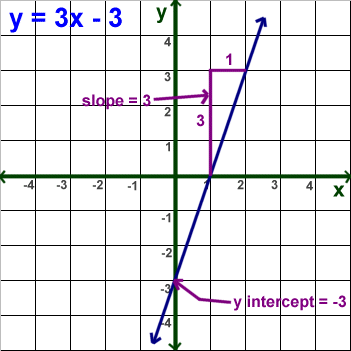# Ms. Minner's Classroom

A Louisville Middle School Classroom

Slope is the direction and steepness of a line. The point at which a line intersects the y-axis is called the y-intercept. The equation a line representing a non-proportional relationship can be written in slope-intercept form, y=mx+b. In the equation y=-6x+2, the slope is -6; and the y-intercept is 2. In the equation y=x-4, the slope is 1; and the y-intercept is -4. In a linear equation, the slope is always the coefficient of a variable (the input variable), and the y-intercept is always a constant.Slope-Intercept Hoop Shoot

My Good Friend Slope Quiz

Stop That Creature Functions

Number Cruncher Function Machine